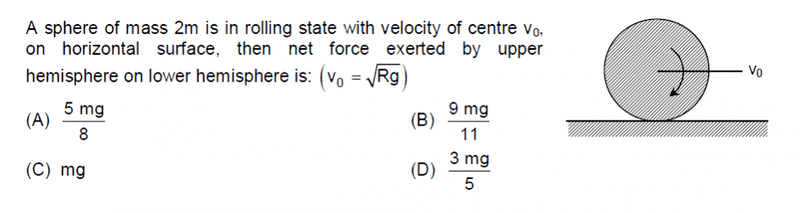# Force exerted on hemisphere

## Homework Statement## The Attempt at a Solution

Using the fact that the sphere is in equilibrium in the 'y' direction , the force exerted by the floor N = 2mg .

Now , if I consider the lower hemisphere then , vertical force exerted by top hemisphere + weight of lower hemisphere = Normal reaction from the floor .

This means , vertical force exerted by top hemisphere on lower = mg

Should this be the total force exerted on the lower hemisphere ?

Thanks

#### Attachments

Last edited:

TSny
Homework Helper
Gold Member
Yes, the sphere as a whole is "in equilibrium in the y direction" since the center of mass of the sphere has no acceleration.

However, does the center of mass of the lower hemisphere have any acceleration?

•Vibhor
However, does the center of mass of the lower hemisphere have any acceleration?

It has centripetal acceleration ω2r ( r=3R/8) directed towards the center of the sphere i.e in +y direction (upwards)

Taking + y to be upwards , 2mg - mg +R = mω2r , gives R = 5mg/8 downwards i.e option A)

Is that ok ??

TSny
Homework Helper
Gold Member
It has centripetal acceleration ω2r ( r=3R/8) directed towards the center of the sphere i.e in +y direction (upwards)

Taking + y to be upwards , 2mg - mg +R = mω2r , gives R = 5mg/8 downwards i.e option A)

Is that ok ??
Yes, I believe that's all correct.

•Vibhor
Yes, I believe that's all correct.

Thank youLast edited: